#Rule 24: Complex FractionsTo manipulate complex fractions, just convert them to simple fractions and follow rules 1 through 23 for simple fractions.Example:
Convert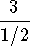to a simple fraction and reduce.can be written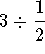. Write 3 as the fraction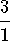. Nowcan be written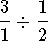. Convert the problem to multiplication by changing the division sign to multiplication and inverting the fraction to the right of the sign.can now be written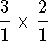and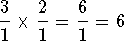. Check your answer with your calculator. Divide 3 by .5 and your answer should be 6. You have just converted a complex fraction to a simple fraction.

### If you want to review another example, click on the word Example.Work the following problems and click on Answer if you want to check the answer and review the solution.

Problem 1:
Simplify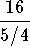and reduce the answer.

Problem 2:
Simplify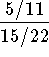.

Problem 3:
Simplify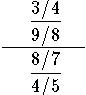[Simple Fractions] [Complex Fractions] [Compound Fractions]
[Decimals] [Percentage] [Rules]

[Algebra] [Trigonometry] [Complex Variables]S.O.S MATHematics home page

Do you need more help? Please post your question on our S.O.S. Mathematics CyberBoard.Author: Nancy Marcus